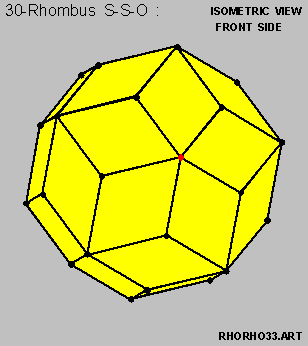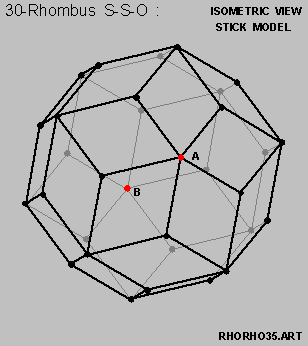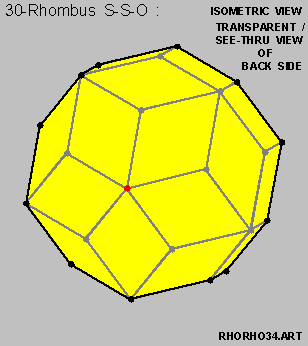The 30-Rhombus Symmetric Solid Object HomePage

# On the 30-Rhombus Symmetric Solid Object

### Summary and Key Findings :

• The [ 30-Rhombus Symmetric Solid Object ] is characterized by :

• the 30 rhombuses being identical to one-another in both shape and size , and

• the centroids of the 30 rhombuses being equi-distant from the center of the said Symmetric Solid Object .• And the [ 30-Rhombus Symmetric Solid Object ] does exhibit Bi-Symmetric , Tri-Symmetric and Penta-Symmetric properties .

#### Table of Content

Part I --- Introduction and Preliminaries Part II --- Constructing the [ 30-Rhombus Symmetric Solid Object ] Section I Introduction Section II Outline on the Method of Construction Section III A Quick Review of the Pentagon Section IV Structural Dimensioning of the Dodecahedron Section V A Quick Review of the Equilateral Triangle Section VI Structural Dimensioning of the Icosahedron Section VII A Quick Review of the Rhombus Section VIII First Attempt at the [ 30-Rhombus Symmetric Solid Object ] Section IX Second Attempt at the [ 30-Rhombus Symmetric Solid Object ] Section X Third Attempt at the [ 30-Rhombus Symmetric Solid Object ] Section XI [ Axis of Penta-Symmetry ] within the [ 30-Rhombus S-S-O ] Section XII [ Axis of Bi-Symmetry ] within the [ 30-Rhombus S-S-O ] Section XIII Five (5) [ Hexa-Symmetric Structures ] within the [ 30-Rhombus S-S-O ] Section XIV Six (6) sets of Parallel Edgess within the [ 30-Rhombus S-S-O ] Section XV [ Axis of Tri-Symmetry ] within the [ 30-Rhombus S-S-O ] Section XVI Co-Planar Axis within the [ 30-Rhombus S-S-O ] Section XVII Reason for calculating the [ Tangent of 9-degrees ] Section XVIII Bringing-in the Tangent Addition Formula Section XIX Setting up the Equation for [ Tangent of 9-degrees ] Section XX Solving the Equation for the [ Tangent of 9-degrees ] Section XXI Constructing the [ 12-Rhombus Symmetric Solid Object ] Section XXII Investigating a different kind of Hexa-Symmetry Section XXIII Concluding Remarks